# How to Calculate XIRR in Mutual Fund [Excel]Many of us invest in mutual funds via SIP (Systematic Investment Plan), which is the best way to take advantage of Rupee cost Averaging. But while calculating returns in SIP, it becomes very important to know What is XIRR, How to calculate XIRR in mutual fund and it’s importance.

XIRR full form is Extended Internal Rate of Return, which is a method to calculate returns on your SIP investments. It becomes very important to calculate XIRR in mutual funds via SIP since we continuously invest via SIP every month, and have occasional redemption from the fund as well.

When we have consistent deposits in mutual funds (or any other investment plan), it becomes difficult to calculate accurate returns using other methods such as absolute returns, CAGR (compounded annual growth rate), etc. and here is where XIRR becomes helpful.

Watch below video to understand how to calculate XIRR in mutual funds with examples.

## How to Calculate XIRR in mutual fund – Video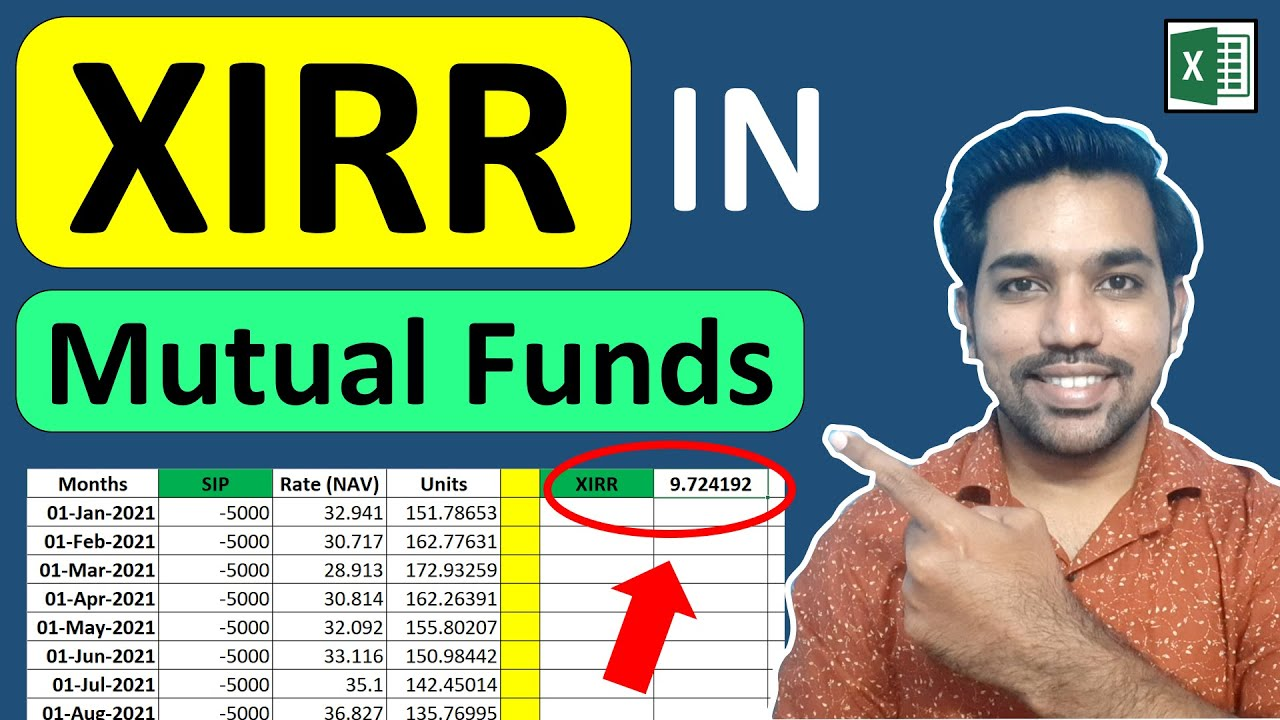Watch more Excel Videos Here

## What is XIRR in Mutual Fund?

XIRR or Extended Internal Rate of Return is a method to calculate returns on your mutual funds.

### Why XIRR? Why not absolute returns or CAGR formula?

Particularly, when we invest via SIP in mutual funds or stocks (which most of us do), or when there is continuous buying of mutual funds in different months of the year and occasional selling as well, we cannot use absolute returns or CAGR returns.

We use XIRR in this case of SIP or continuous buying or selling of mutual funds.

It takes into consideration different dates of buying and selling of mutual funds which is the perfect example in SIP, in which case absolute returns or CAGR is of less use for us.

In below sections, we have covered when to use all these ways of calculating returns in mutual funds.

### When to use Absolute Returns?

Absolute returns in mutual funds can be used when we do a lump sum investment in mutual funds (or stocks), and then sell them within one year. In this case we find difference between selling and buying price and get the absolute returns as percentage.

Please note that while buying and selling mutual funds or stocks within a year, you may attract short term capital gains tax!

So absolute returns formula is:

Absolute returns = (Selling Price – buying price) * 100 / buying price

In above formula, if selling price is greater than buying price of mutual funds, we’ll get positive absolute returns or else if selling price is less than buying price of mutual funds, we’ll get negative returns.

### When to use CAGR?

Now as seen above, we use absolute returns while buying and selling mutual funds within one year.

But when we do lump sum investment in mutual fund and sell them after 2 or 3 years, we have to use CAGR to get annual growth rate on our investment.

And below is the formula for calculating CAGR:

CAGR = [(Final value / Beginning value) ^ 1 / t] – 1

where:

So we use CAGR returns formula to get annual growth rate on multiple years of investments.

### When to use XIRR?

As mentioned above, during lump sum investment and withdrawal we either use absolute returns or CAGR.

But in case we are investing in mutual funds via SIP, we have to use XIRR to get accurate returns.

Read on to know more about importance of XIRR while investing via SIP.

## XIRR importance in SIP

Below are the cases when we should use XIRR formula:

• Calculating accurate returns over a specific period of time on SIP
• Returns calculation when there is irregular investments and withdrawals
• Calculating returns when lump sum amount is redeemed after SIP investments
• Returns calculation when withdrawing systematically (SWP) after lump sum investment

Watch this video to know returns calculation on SWP (Systematic Withdrawal Plan) using Excel:

### SWP Returns Calculation in Excel – Video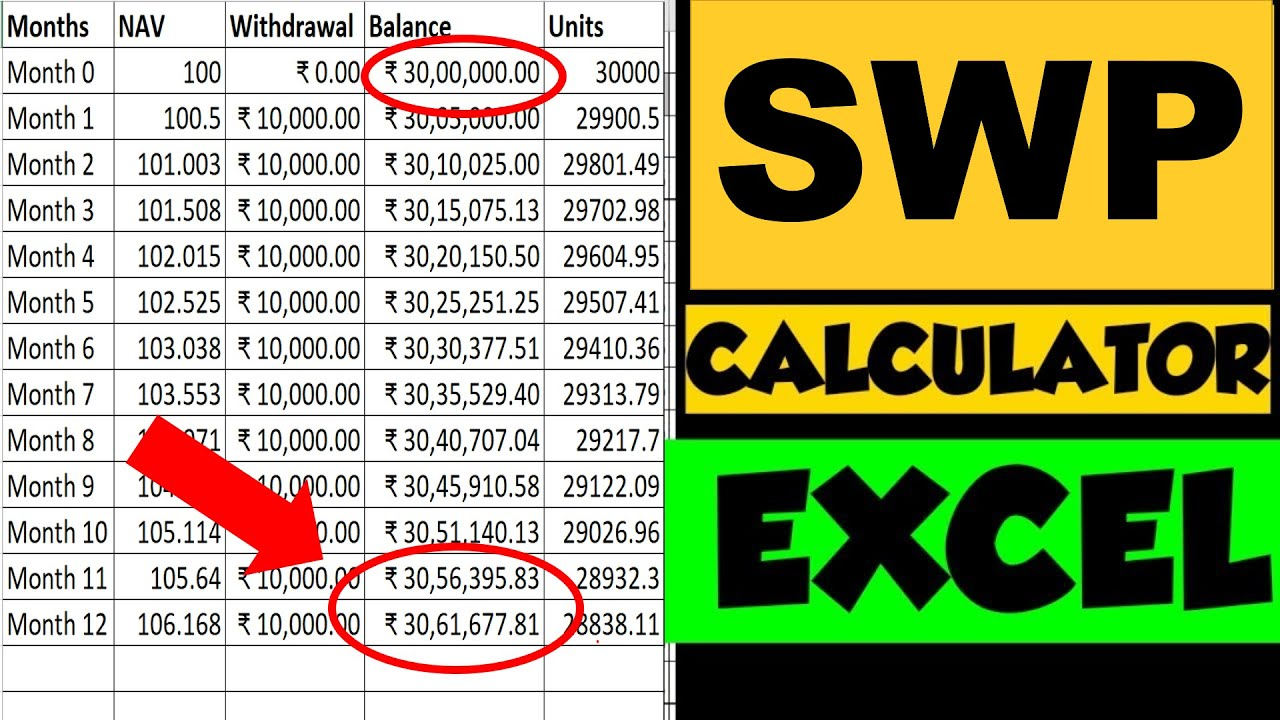Watch more Excel Videos Here

Let us now see the formula for XIRR in Excel.

## What is the Formula for XIRR?

We can easily use the formula for XIRR in excel and calculate returns on our SIP. Following is the XIRR formula in excel:

= XIRR (values, dates) * 100

where:
values = list of values of SIP Investments – negative values for deposits and positive values for withdrawals
dates = list of dates on which amounts were deposited and withdrawn

Below is the image of how XIRR formula looks like in excel:

As seen in above image, B column has values – negative values as deposits as this indicates debit amount and positive amount as withdrawals (or current investment value) which indicates that this amount will be credit if withdrawn right now.

A column contains the list of specific dates with required deposits and withdrawals (or current investment value).

## How to Calculate XIRR in Mutual Fund Manually?

The good way to calculate XIRR or extended internal rate of return on mutual funds is via Excel formula of XIRR.

Let us check this with the help of example.

### XIRR Calculation Example

Let’s say we invest Rs. 5000 every month in a mutual fund in 2021, between 1st January 2021 to 1 December 2021. Also, we have tracked the NAV values of mutual funds for these dates of investments.

Below is the image with calculated number of units accumulated every month for 1 year:

Notice that we have used negative values for SIP deposits, as it indicates debit amount every month. Also we have calculated units in last column based on formula:

Units = Amount / NAV

We can now add all units in last column, and by using the latest NAV, if we sell all these units, we get Rs. 65,087 as the final value (for units = 1744.76 * NAV = Rs. 37.304)

Below is the image for this final value calculation:

So if we calculate, our total deposits is Rs. 60,000 for 12 months and the final value is Rs. 65,087, which is higher than the total deposits.

This means that XIRR returns will be positive in this case. Let us calculate XIRR!

By using the formula of XIRR in excel:

= XIRR (values, dates) * 100

let’s put this formula in excel in our example:

So as seen, we get 16.65% XIRR returns for this example.

Similarly by following above steps, you can calculate XIRR on your mutual fund investments.

More Recommended Books Here

## What is Good XIRR Percentage?

Now what can be the good XIRR returns on the investments.

Anywhere between 10% to 12% can be considered good XIRR percentage on average. Getting more than 12% XIRR percentage will be a great investment.

ALSO READ: Income Tax on SIP Maturity [Excel]

## Is XIRR same as CAGR?

As mentioned above, CAGR (Compounded Annual Growth Rate) is used while calculating the compound rate of growth over multiple years for a lump sum investment.

Whereas, XIRR is the average rate earned by each and every cash flow invested during the period. So every cash flow or deposits is considered to calculate XIRR percentage.

And this is the difference between CAGR and XIRR.

XIRR can be treated as aggregation of multiple CAGR.

## XIRR compounded daily?

XIRR is used to calculate returns based on various dates, which can be daily as well. But this does not mean that XIRR compounds daily.

XIRR still compounds on annual basis considering the dates and values of investments.

If compared with the compounding effect of PPF (Public Provident Fund), we will get almost same results with XIRR in SIP thus proving that XIRR compounds annually.

ALSO READ: Compounding in SIP using Excel

## Is XIRR per annum?

Yes XIRR percentage calculation gives us per annum rate.

Since the investments made are regular on same date of every month or irregular on different dates of month, XIR considers all these factors to give XIRR percentage per annum.

## Does XIRR work for less than 1 year?

Yes XIRR can work for less than 1 year as well. But it is best to find XIRR percentage on investments above 1 year to get annualized returns percentage.

For calculating returns on less than a year, mention appropriate dates and values of investments (negative numbers for deposits and positive numbers for withdrawals).

Some more Videos

## Income Tax Calculation FY 2023-24 Video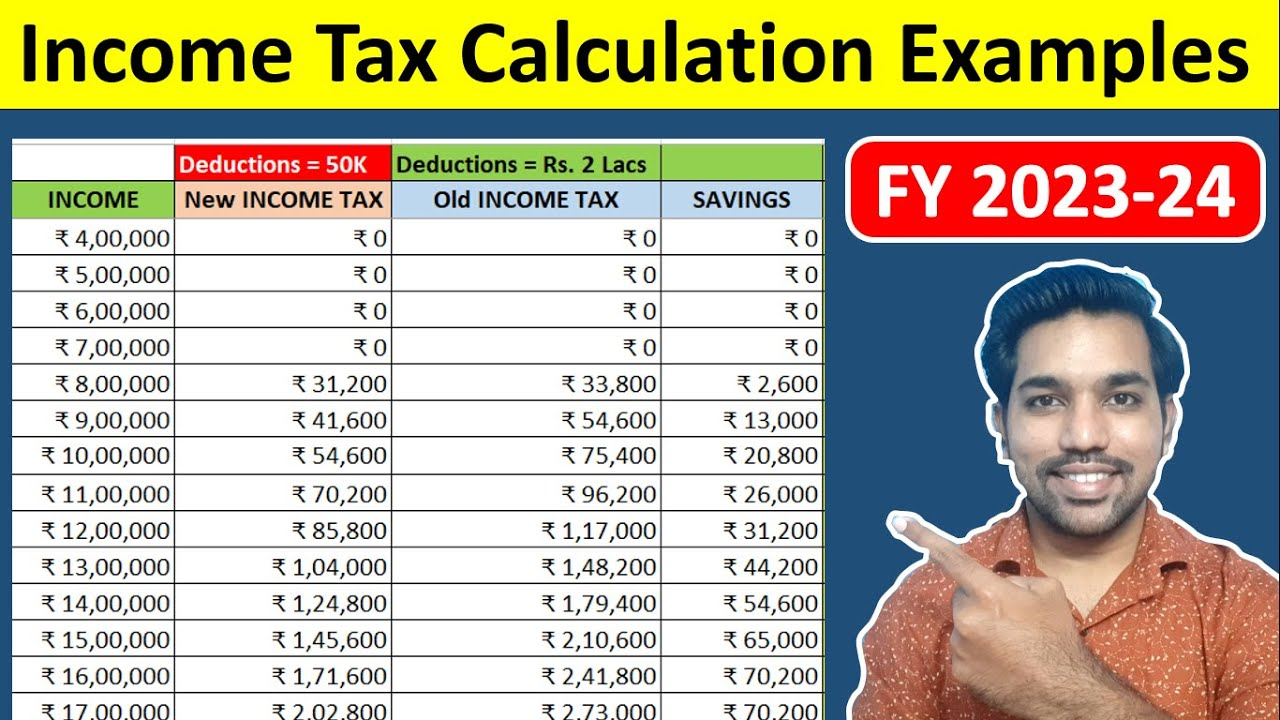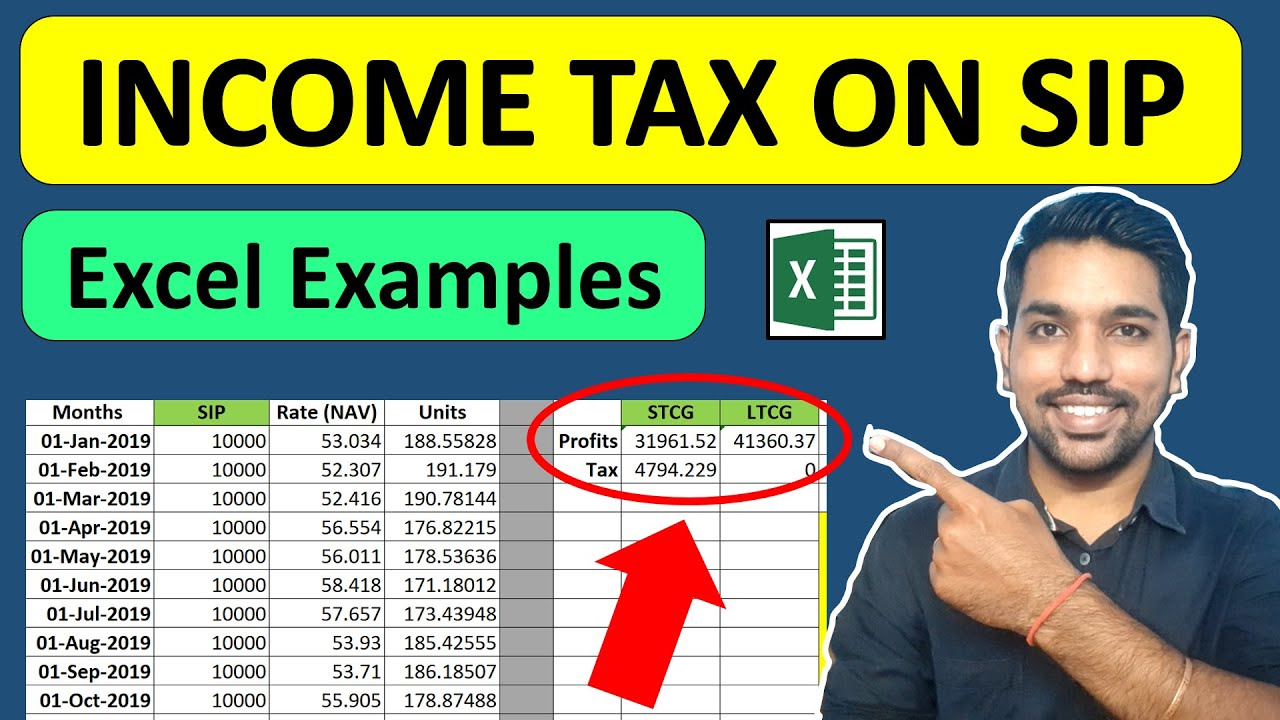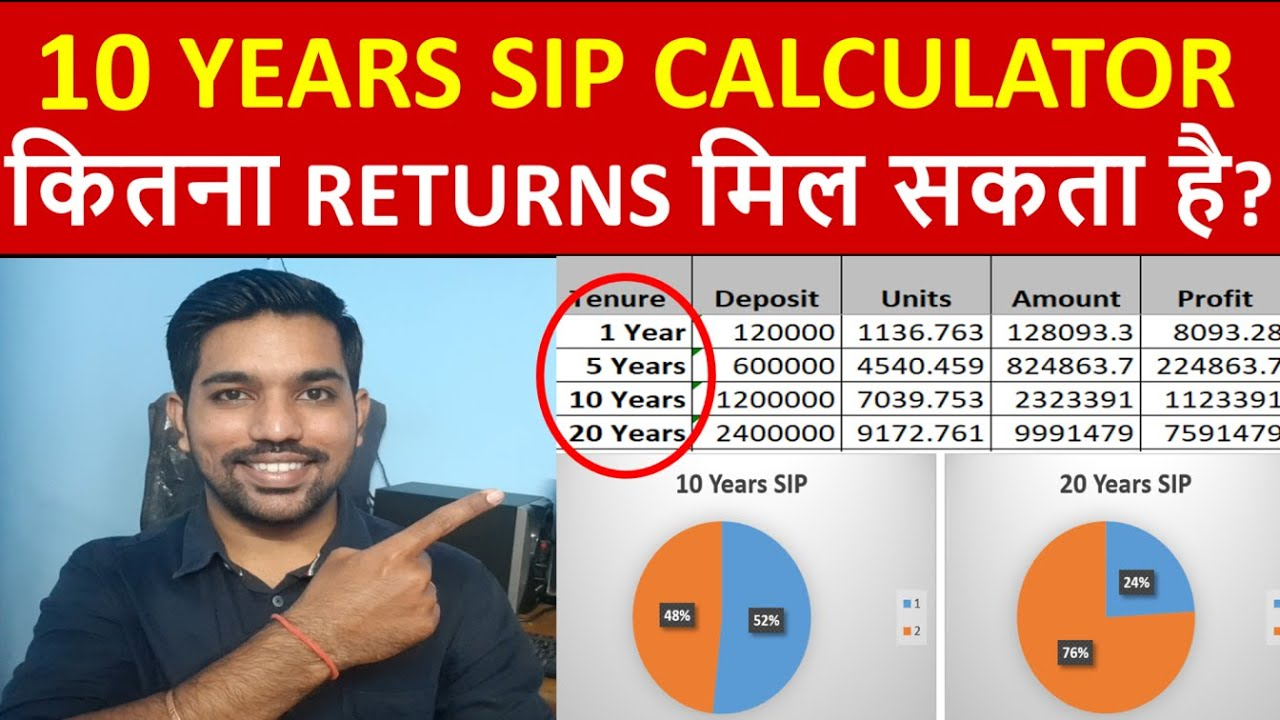## You can Donate Too!

Found this Helpful? DONATE any amount to see more useful Content. Scan below QR code using any UPI App!

UPI ID: abhilashgupta8149-1@okhdfcbank

Verify that you are “Paying Abhilash Gupta” before making the transaction so that it reaches me. It makes my Day 🙂

Thank you for Donating. Stay Tuned!

## Income Tax Calculator App – FinCalC

For Income Tax Calculation on your mobile device, you can Download my Android App “FinCalC” which I have developed for you to make your income tax calculation easy.

### What you can do with this mobile App?

• Calculate Income Tax for new FY 2023-24 and previous FY 2022-23
• Enter estimated Investments to check income tax with Old and New Tax Regime
• Save income tax details and track regularly
• Know how much to invest more to save income tax
• More calculators including PPF, SIP returns, Savings account interest and lot more